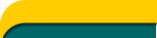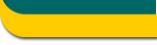+ Text Only Site
+ Non-Flash Version
+ Contact GlennAbout Rockets Educator Section Rocket Safety The Simulators Install 3D Simulator Home

START YOUR JOURNEY - LAWS OF MOTION

Newton’s Laws of Motion ChallengeYou Chose : 2. The Law of Proportionality: “The acceleration of an object is directly proportional to the net force and inversely proportional to its mass.” This can be expressed in equation form: Force = (Mass) x (Acceleration)

You chose wisely!

When a net, or excess, force is applied to an object, it will accelerate in the direction of the force. In physics we say that the acceleration is directly proportional to the force.

Of course, the more massive the object, the slower it will accelerate. In this case, we say that the acceleration is inversely proportional to the mass (as mass increases, the acceleration decreases, and vice-versa).

We write this equation as:

Force (thrust) = Mass x Acceleration

Or we can write it as:

Acceleration = Force ÷ Mass

Example: The force is 25.0 lbs of thrust. The rocket has a mass of 1.400 kilograms. Calculate the rocket's acceleration if it moved HORIZONTALLY (we don’t want to fight gravity yet), on some frictionless surface.

Solution: First we need to change pounds of thrust into the metric equivalent of pounds…a unit called Newtons. We do this by multiplying 4.45 Newtons per pound times the number of pounds of force, so….25.0 lbs x 4.45 Newtons/lb = 111.3 Newtons of metric force.

 Acceleration = (111.3 Newtons) ÷ (1.4 kilograms) Acceleration = 79.5 Newtons per kilogram = 79.5 meters per second each second

That means that the rocket’s speed increases by 79.5 meters per second every second.

If we fire the rocket vertically, its acceleration will be a bit less since it will struggle against gravity. That calculation is:

Acceleration = Force ÷ Mass

If the rocket moves vertically, there are TWO forces: the upward thrust of 25 pounds, or 111.3 Newtons, and the downward pull of gravity, which is the rocket’s weight.

First, what does the rocket weigh?
The rocket has a mass of 1.40 kilograms. Any object’s weight is found by multiplying its mass times the force of gravity. On the surface of the Earth, the force of gravity is 9.80 Newtons for every kilogram of mass (written as 9.8 N/kg).

So,

 Weight = mass x gravitational force Weight = (1.40 kilograms) x (9.80 Newtons per kilogram) Weight = 13.7 Newtons

Let’s use the weight in the acceleration equation:

 Acceleration = Force ÷ Mass Acceleration = (111.3 Newtons – 13.7 Newtons) ÷ (1.4 kilograms)

Notice we SUBTRACT the weight. It’s holding our rocket back!

Acceleration = 69.7 meters per second each second.

>> Back to the Acceleration page

 Any comments, concerns, or questions should be addressed to: Developer: David Mazza     Responsible NASA Official: Jo Ann Charleston+ Inspector General Hotline + Equal Employment Opportunity Data Posted Pursuant to the No Fear Act + Budgets, Strategic Plans and Accountability Reports + Freedom of Information Act + The President's Management Agenda + NASA Privacy Statement, Disclaimer, and Accessibility CertificationEditor: Tom Benson NASA Official: Tom Benson Last Updated: May 13 2021 + Contact Glenn